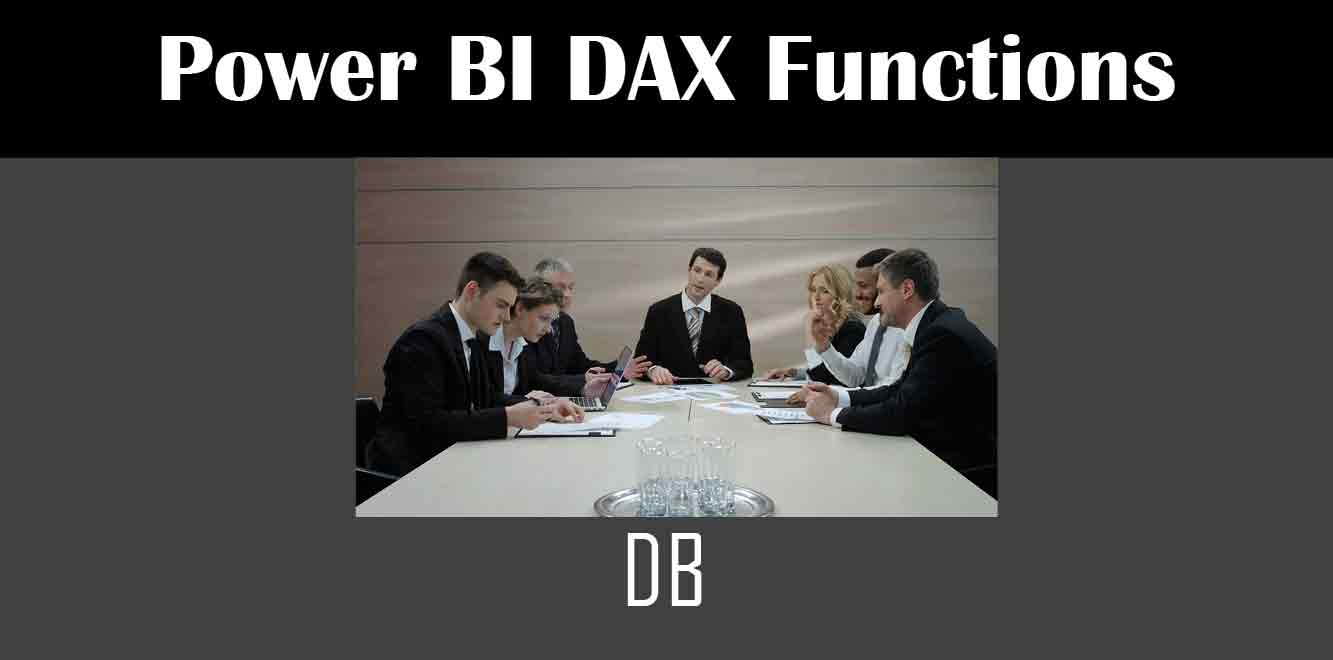# DB

## YThe DB function in DAX is used to calculate the depreciation of an asset over a specified period of time. The function takes four arguments:

– Cost: The initial cost of the asset

– Salvage: The value of the asset at the end of its useful life

– Life: The useful life of the asset in years

– Period: The period for which you want to calculate the depreciation

The formula for DB is as follows:

`DB(Cost, Salvage, Life, Period)`

Let’s take a closer look at each of the arguments.

## Cost

Cost is the initial cost of the asset. This can be the purchase price of the asset, plus any additional costs associated with acquiring and preparing the asset for its intended use. For example, if you purchase a new machine for your factory, the cost would include the purchase price of the machine, as well as any shipping, installation, or training costs.

## Salvage

Salvage is the value of the asset at the end of its useful life. This is also referred to as the “residual value” of the asset. For example, if you purchase a car for your business and plan to use it for five years, the salvage value would be the estimated value of the car at the end of the fifth year.

## Life

Life is the useful life of the asset in years. This is the estimated number of years that the asset will be used before it is no longer useful. For example, if you purchase a computer for your business and plan to replace it every three years, the useful life would be three years.

## Period

Period is the period for which you want to calculate the depreciation. This can be a single period, such as a month or a quarter, or it can be a range of periods, such as the first year or the first five years of the asset’s useful life.

Now that we understand the arguments for the DB function, let’s look at an example.

Suppose you purchase a machine for your factory for \$50,000, and you estimate that it will have a useful life of 10 years with a salvage value of \$5,000. You want to calculate the depreciation expense for the first year of the machine’s useful life. Using the DB function, the formula would be:

`DB(50000, 5000, 10, 1)`

The result of this formula would be \$5,000, which represents the depreciation expense for the first year of the machine’s useful life.

In conclusion, the DB function in DAX is a powerful tool for calculating the depreciation of assets over a specified period of time. By understanding the arguments for the function and how to use it in a formula, you can gain valuable insights into the financial performance of your business.

Subject